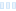Hi, this is my first topic here and I want to know how do I make matrices in Ultra Fractal.

Thanks!

Hi, this is my first topic here and I want to know how do I make matrices in Ultra Fractal. Thanks!

0

Matrices are called arrays. See the help file for a brief description.

Matrices are called arrays. See the help file for a brief description.

0

Matrices are called arrays. See the help file for a brief description.

I never thought of that before. Could be worth a try. Maybe an example with a two-variable Newton fractal could give me a template?

&gt;Matrices are called arrays. See the help file for a brief description. I never thought of that before. Could be worth a try. Maybe an example with a two-variable Newton fractal could give me a template?

0

It's harder because there is no library of matrix manipulations, like matrix-vector multiplication or solving linear equations. I've mainly seen arrays used for remembering intermediate results for later use, such as
complex zsave[#maxiter+1]
...
zsave[i] = pz
...
And do something with the array at the end.

It&#039;s harder because there is no library of matrix manipulations, like matrix-vector multiplication or solving linear equations. I&#039;ve mainly seen arrays used for remembering intermediate results for later use, such as complex zsave[#maxiter+1] ... zsave[i] = pz ... And do something with the array at the end.

0

Since the addition of functions/classes in UF5, it's possible for
someone to write a library of matrix/vector manipulation functions. So
this doesn't have to a built-in feature.

It turns out we can make a library of matrix/vector multiplication functions. So, where do I start?

While function declarations in formulas are useful, you cannot access them from another formula. To be able to re-use code in multiple formulas, you need to use classes instead.
A class is a declaration of a set of variables and functions that operate on these variables.
An object is an instance of its class. This class is the type of the object, just like int is the type of an integer variable.
The variables of a class are called fields.
The functions of a class are called methods. Both fields and methods are also known as the members of a class.
Classes are declared in formula files, or in plug-in library files with the .ulb file extension. A class looks a bit like a regular formula entry, but the entry identifier is preceded by the class keyword. Here is an example of a simple class that represents a point with integer coordinates:

``````class Point {
public:
func Point(int aX, int aY)
x = aX
y = aY
endfunc

float func distance(Point p)
; Returns the distance to the second point p.
return sqrt(sqr(p.x - x) + sqr(p.y - y))
endfunc

int x
int y
}
``````

This class, named Point, contains a constructor, which is a special function that initializes an instance of the class. The constructor always has the same name as the class. Also, there is an additional method called distance, and two fields called x and y that store the coordinates of the point.

This could come in handy.

&gt; Since the addition of functions/classes in UF5, it&#039;s possible for someone to write a library of matrix/vector manipulation functions. So this doesn&#039;t have to a built-in feature. It turns out we can make a library of matrix/vector multiplication functions. So, where do I start? &gt; While function declarations in formulas are useful, you cannot access them from another formula. To be able to re-use code in multiple formulas, you need to use classes instead. A class is a declaration of a set of variables and functions that operate on these variables. An object is an instance of its class. This class is the type of the object, just like int is the type of an integer variable. The variables of a class are called fields. The functions of a class are called methods. Both fields and methods are also known as the members of a class. Classes are declared in formula files, or in plug-in library files with the .ulb file extension. A class looks a bit like a regular formula entry, but the entry identifier is preceded by the class keyword. Here is an example of a simple class that represents a point with integer coordinates: ```` class Point { public: func Point(int aX, int aY) x = aX y = aY endfunc float func distance(Point p) ; Returns the distance to the second point p. return sqrt(sqr(p.x - x) + sqr(p.y - y)) endfunc int x int y } ```` &gt; This class, named Point, contains a constructor, which is a special function that initializes an instance of the class. The constructor always has the same name as the class. Also, there is an additional method called distance, and two fields called x and y that store the coordinates of the point. This could come in handy.

0

Note that we already have a Vector class in the common.ulb file. If you were to add a matrix library (in your own ulb file), it would be handy to use that Vector class.

Note that we already have a Vector class in the common.ulb file. If you were to add a matrix library (in your own ulb file), it would be handy to use that Vector class.

Ultra Fractal author

1
87
views
5
replies
3
followers
live preview
Enter at least 10 characters.
WARNING: You mentioned %MENTIONS%, but they cannot see this message and will not be notifiedSaving...
Saved
All posts under this topic will be deleted ?
Pending draft ... Click to resume editing